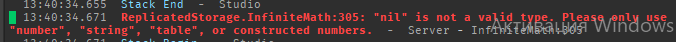# Printing a number prints it as 0, but comparing says a number is table(InfiniteMath module issue ig)

1. What do you want to achieve? Keep it simple and clear!
Topic says everything

2. What is the issue? Include screenshots / videos if possible!

3. What solutions have you tried so far? Did you look for solutions on the Developer Hub? I tryed reconverting number with InfiniteMath module(one that helps bypassing 1.8e308 limit) , but no point why print says value is 0 and comparing says this is table, i have no point why.

Code

``````...
if amount < InfiniteMath.new(80) then
print(amount)
local Models = game:GetService("ServerStorage").Models
if amount > 0 then
for i=1,amount do
local Clone = Models.Photon:Clone()
Clone.Parent = workspace.FirstMap.Photons
end
else
local Models = game:GetService("ServerStorage").Models
for i=1,80 do
local Clone = Models.Photon:Clone()
Clone.Parent = workspace.FirstMap.Photons
end
end
end
end
...
``````

Scroll down to the end of this section and you will find why.

1 Like

From looking at the documentation for InfiniteMath (GitHub - KdudeDev/InfiniteMath: InfiniteMath is a Roblox LuaU module that allows you to surpass the number limit (1 * 10^308))

edit: Jqck also found it in the documentation. I’ll see if I can find a workaround mentioned, cause it also says

Numbers constructed from InfiniteMath supports arithmetic operators `(+, -, *, /, ^, %)` with constructed numbers and normal numbers, and comparison operators `(<, >, <=, >=, ==, ~=)` with other constructed numbers. InfiniteMath also has support for OrderedDataStores.

I know about that. Maybe you didn’t understand topic correctly. As i mentioned i was reconverting value back to default one and it still outputs same error. And print says this is default number(because it printing number and not table)

``````if amount < InfiniteMath.new(80) then
print(amount)
local Models = game:GetService("ServerStorage").Models
if InfiniteMath.round(amount) > 0 then
``````

give absolutely same problem

From looking more at the documentation:
It may be possible to replace

``````if amount < InfiniteMath.new(80) then
``````

with

``````if InfiniteMath.new(amount) < InfiniteMath.new(80) then
``````

to get it to work

Not this line give error. Amount already converted to infinite math table

``````if InfiniteMath.round(amount) > 0 then
``````

this one

how is the value of amount set?

``````local amount = InfiniteMath.new(PlayerManager.GetValue(player, "Photons", "Current"))
ServerFunctions:SpawnPhoton(player, amount, true)
if amount > InfiniteMath.new(0) then
ClientFunctions:TextTransparent(player.PlayerGui.GameGUI.Count, "decrease", 150)
end
``````

Also why print says it just 0 instead of table, i’m not getting it

InfiniteMath.new(0)

If you try that sorta thing where the error occurs, does it resolve the issue?

Also found this function here in the documentation

If you call this function upon the InfinteMath.new() number, it may work

Ah i can’t really see. This is some strange thing, InfiniteMath tables don’t go though function. Other spawn function(which is increasing value, not one which loading(frm which topic is done) now says amount is not a table and cap is table. But i converted amount to table in server script and then sent it to module. So i should compare table to table there, but Lua thinks amount is value somehow

This is same as amount:Reverse(). It gives me nil value(can’t convert 0 ig)

Ah wait nevermind. I had 2 amounts in that spawn script, forgor to convert one of them. Really quick will see if InfiniteMath.new(0) will solve thisbruh

What was the exact line of code that caused that, plus how any variables called in that line are assigned?

There’s this inbuilt feature called `metatables` which just basically makes a regular table run on steroids. Using metatables, you can assign certain `metamethods` (usually functions) to a table which give it extended functionalities. One of such metamethod is `__tostring` which is function that is called whenever you print the table. Now if we look into the InfiniteMath module, you will see that the table `InfiniteMath` has a metatable attached to it called `Number`, and if we look into its metamethods, you will find:

``````function Number.__tostring(self)
return self:GetSuffix(true)
end
``````

where `:GetSuffix` is the function which return a `string` which is the value of the number after doing some calculations (in your case 0). There are also other arithmetic metamethods like
`__eq`, `__add`, `__sub` which are called when you do a == b, a + b and a - b, respectively. That’s why you can only perform such calculations between two InfiniteMath objects because the system is designed to do as such.

1 Like

Forget about that. I forgot to finish gain formula(i’m reworking it gain, lemme just make 1 instead)

No this won’t help. Because even if now i have more than 1 photon next line

``````	for i=1,amount do
``````

will have same error(number and table).

``````	for i=1,InfiniteMath.Reverse(amount) do
``````

this line gives nil. This method

``````	for i=1,InfiniteMath.round(amount) do
``````

gives same error(number and table)
Btw photon amount is 2

Can you recap what’s your problem in brief and send the code?